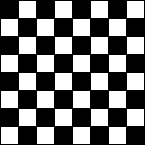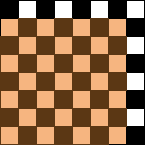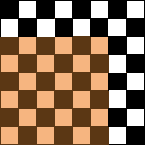Name: tchilco Question: How many squares are on a chessboard? Hi, A chessbaord is 8 by 8 and hence there are 8x8 = 82 possibilities for the placement of a 1 by 1 square on a chessbaord.To count the number of ways you can place a 2 by 2 square on a chessbaord, watch where the lower left corner of the 2 by 2 square can go. There are 7x7 = 72 possibilities for the placement of the lower left corner of a 2 by 2 square on a chessboard.Likewise to count the number of ways you can place a 3 by 3 square on a chessbaord, watch where the lower left corner of the 3 by 3 square can go. There are 6x6 = 62 possibilities for the placement of the lower left corner of a 3 by 3 square on a chessboard.You should be able to complete the problem now. Cheers, Penny and Claude Go to Math Central